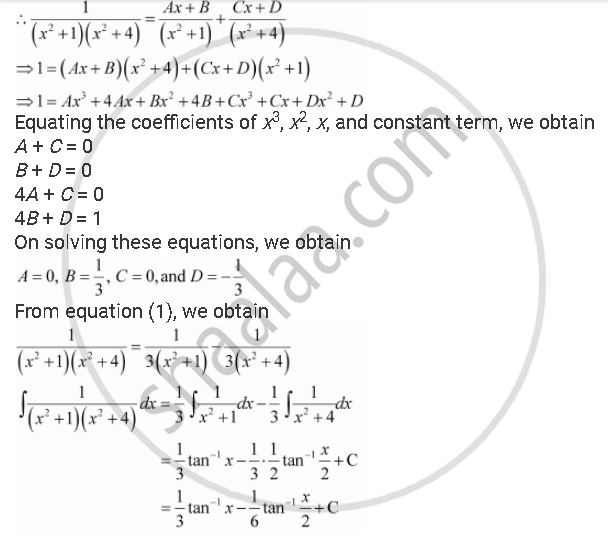Share
Notifications

View all notifications
Books Shortlist
Your shortlist is empty

# Solution for Integrate the Functions 1/((X^2 + 1)(X^2 + 4)) - CBSE (Science) Class 12 - Mathematics

Login
Create free account

Forgot password?
ConceptIntegration as an Inverse Process of Differentiation

#### Question

Integrate the functions  1/((x^2 + 1)(x^2 + 4))

#### SolutionIs there an error in this question or solution?

#### Video TutorialsVIEW ALL 

Solution Integrate the Functions 1/((X^2 + 1)(X^2 + 4)) Concept: Integration as an Inverse Process of Differentiation.
S